## Orbits in 2D

Orbital Motion: motion of a particle with a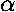/r2 central force applied. In intro physics the topic was the motion of planets under the influence of the Sun's gravitational force...orbital mechanics. In quantum mechanics the topic is the motion of an electron under the influence of the electrostatic attractive force of the nucleus...atomic physics. Equivalent equations for the force (and hence "the same" as far a physics goes) but quite different distance scales.

Planets move in ellipses with the Sun at one focus. Ellipses can be described in terms of their semi-major axis, a, (basically the longest radius) and their eccentricity (basically how squashed the ellipse is: e=0 is a circle, a fully squashed ellipse looks like a line and has e=1).

r=a(1-e2)/(1+e cos(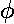)); rmin= a(1-e); rmax=a(1+e), the polar angle from closest approach is given the odd name: true anomaly. The timing of the motion (i.e., when the planet or electron has a particular) is a bit complex. The game is to express the true anomaly () in terms of the eccentric anomaly (u) and then find an expression relating time and the eccentric anomaly. For nearly circular orbits it turns out that the true anomaly, the eccentric anomaly, and the mean anomaly (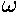t) are all approximately equal to each other. I apologize for this archaic nomenclature, but physics is stuck with these names. Below find the geometric construction that relates the true anomaly and the eccentric anomaly, the formula relating these two, and the formula relating time and the eccentric anomaly.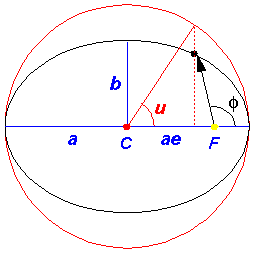In the above picture, the yellow dot represents the Sun (or a nucleus); it is at a focus (F) of the ellipse. The red parts of the diagram have to do with the geometric construction for the eccentric anomaly which is measured from the center (C) of the ellipse. There is nothing physically at the center of the ellipse, this is all just part of a geometric construction. The blue parts of the diagram relate the semi-major axis (a) and the distance between the ellipse center and the focus (ae). The semi-minor axis, b, can be related to the semi-major axis and the eccentricity:

b = a(1-e2)½

The black ellipse is the orbit of the particle, i.e., the set of positions the particle will traverse during a period (T=2/).

It is most convenient to express the position of the particle in terms of u:

r = ( a(cos(u)-e), a (1-e2)½ sin(u) )

In Mathematica we can easily display the orbit:

```a=1; e=N[1/Sqrt]
ParametricPlot[{a (Cos[u]-e),a Sqrt[1-e^2] Sin[u ]},{u,-Pi,Pi}]
```
But we have to work to find the time dependence of the motion. Here we divide the period up into 50 "tics". We must first create storage locations for our u values and corresponding position vectors:
```uu=Table[0,{51}]
rr=Table[{0,0},{51}]

Do[
uu[[i]]= u /. FindRoot[u-e Sin[u] == .04 Pi (i-26),{u,.04 Pi (i-26) }] ;
rr[[i,1]]=a (Cos[uu[[i]]]-e) ;
rr[[i,2]]=a Sqrt[1-e^2] Sin[uu[[i]]] ,
{i,51}]

```
The second part of the above is fairly complicated and has to do with solving Kepler's equation:We seek u values that correspond to equally spaced times. Thus we partition one full cycle:
-<t <into little intervals:ti =(i-26)/25

as i runs from 1 to 51,ti runs through one period. Finding the u that corresponds to each instant ti, requires solving Kepler's equation. Kepler's equation cannot be solved with algebra: we must numerically find the ui that produces the proper ti...that's what the FindRoot is doing. Once we have found our ui that satisfies Kepler's equation, we substitute it in ("/.") for u and save it in uu[[i]]. From uu[[i]] we can calculate the corresponding

rx=rr[[i,1]]
ry=rr[[i,2]]

We can then plot these points:

```ListPlot[rr]
```
We can combine the dot-plot with the orbital curve with a Show (say the ParametricPlot was Out and the ListPlot was Out:
```Show[%10,%6,AspectRatio->Automatic]
```

If you want you can figure out the variousi:

```pp=Table[0,{51}]
Do[
pp[[i]]=N[2 ArcTan[ Cos[uu[[i]]/2], Sqrt[(1+e)/(1-e)] Sin[uu[[i]]/2] ] ],
{i,51}]
```

The velocity vector is, of course, changing as the position vector is changing. The set of velocities the particle will have during a period is called the hodograph; it's just like an orbit, but for velocity rather than position. The hodograph is surprising: it's just a circle, but the center of the circle is not v=0.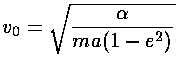Note that at closest approach to the "Sun" (=0) the speed is a maximum and the velocity points in the y direction, whereas at the far point (=180°) the speed is a minimum and the velocity points in the -y direction. As the eccentricity approaches 1, the maximum speed gets arbitrarily large and the minimum speed approaches zero.

We could now use Mathematica to make plots of the velocity vector:

```vxy=Table[{-Sin[pp[[i]]],Cos[pp[[i]]]+e},{i,51}]
ListPlot[%,AspectRatio->Automatic]
ParametricPlot[{-Sin[u ],(Cos[u]+e) },{u,-Pi,Pi}]
Show[%,%%,AspectRatio->Automatic]
```

The above figures have been drawn with a rather large eccentricity: e=.707. For eccentricities similar to those of the planets it would be hard to distinguish the elliptical orbit from a circle (although for some planets--like Mars--the position of the Sun would look noticeably "off-center"...because the Sun is at a focus rather than the center).

If the (attractive) radial force is given by: Fr=-/r2, the potential energy is U(r)=-/r, so that the minus derivative of U is the force. Notice that just like the spring (U(x)=½k x2, which also attracts to the origin), the potential energy is ever smaller as you approach the origin. Unlike the spring, this potential energy is numerically negative (i.e., U(r)<0). That is a result of our choice of additive constant. For the spring we choose the potential to be zero at the origin, forcing the potential to be arbitrarily big as x approaches infinity. For this problem we take the potential energy to be zero as r approaches infinity, so the potential at the origin must be infinitely less, i.e., negative infinity. The seemingly over-complex geometric description of motion:can, with some work, be seen to solve the equation of motion: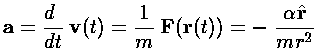Start by noting that the velocity vector is made of a constant vector (ev0j) added to the red rotating vector. Taking the time derivate removes the constant from consideration, so the acceleration vector must be tangent to the circle--i.e., rotated 90° from the red radial vector, which is itself rotated 90° from r (note that the zero forwas the x-axis for the orbit and the y-axis in the hodograph). Thus the acceleration vector is in the right direction: -r. The magnitude of the acceleration vector is just: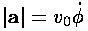is related to the (constant) angular momentum (L) [which we can evaluate for example, at the near point].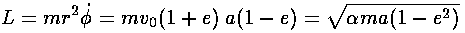Solving the above forand substituting into our equation for the magnitude of the acceleration vector, yields: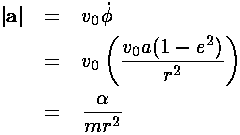Note in passing that if the angular momentum is zero, then e=1. The circular orbits (e=0) have the maximum possible angular momentum for a given semi-major axis a, that is for a given energy E because there is a simple relation between the semi-major axis a and E: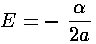Note that the energy is negative; the positive kinetic energy equals (on average) one half the absolute value of the potential energy (the Virial theorem again!) and the result is that the total energy is ½ the average potential energy.

Here is another way of presenting the results: plots displaying the position of the particle on the orbit and the velocity of the particle on the hodograph at a series of equally spaced times.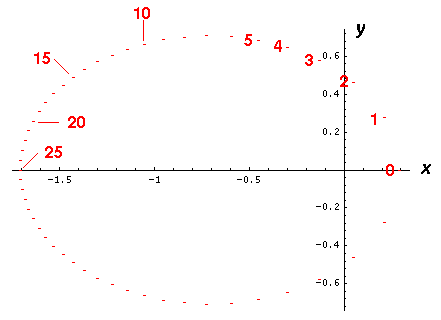The above is a plot of the (x,y) location of a electron (or planet) at successive increments of time (tics) Note the when the electron (planet) is close to the nucleus (or Sun), the particle seems to move a great distance between tics, whereas when the particle is is far from the origin, it seems to move only a little between tics. This is exactly what you would expect for a particle conserving angular momentum (i.e., following Kepler's second law of constant areal velocity).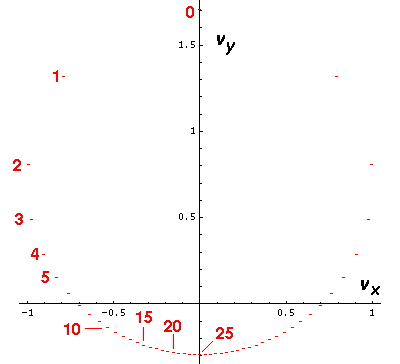The above is a plot of the (vx,vy) velocity of a electron (or planet) at successive increments of time (tics) Note the when the electron (planet) is close to the nucleus (or Sun) (e.g., near tic 0) the velocity seems to change quite a bit between tics, whereas when the particle is is far from the origin (e.g., near tic 25), the velocity seems to change only a little between tics. This is exactly what you should expect for a 1/r2 force.

Note that the motion is confined to a plane so we can display the results on a flat screen.

If we ignore the angular part of the motion, we can make plots of the the radial velocity vs. time (in tics), the distance from the origin (r) vs. time, and the speed (v) vs. time: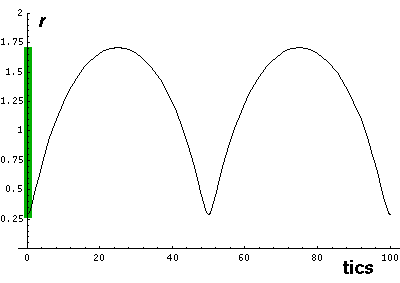Note that r seems to "bounce" off the minimum radius rmin. The motion is in fact quite similar to the bouncing falling ball. Here vr changes quickly (but not instantaneous) between negative (falling) and positive (rising) values. The green area on the r plot shows the classically allowed region.

Note that while r does range between two values: rmin= a(1-e) & rmax=a(1+e), these turning points are a bit different from 1-d turning points as v is never zero. We can, however, threat our 3-d (reduced to 2-d) motion as a 1-d motion in an effective potential (see for example, Marion p.301, Barger & Olsson p.138, Goldstein p.61) which includes the centrifugal potential: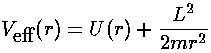The above diagram plots various the energies involved as a function of r. The line representing "Total Energy" is flat, because total energy is conserved (i.e., is constant). Total energy is the sum of kinetic energy and potential energy. We can decompose kinetic energy (½mv2) into two pieces by using the Pythagorean theorem to decompose v2 into the sum of two terms: radial velocity squared (vr2) and the component of velocity perpendicular to r ("transverse" velocity) squared. The "transverse" kinetic energy, can be calculated from the angular momentum and r...the result is the centrifugal potential. The centrifugal potential has been added to the 1/r potential; the result is plotted in red. Thus total energy is the sum of the effective potential and the "radial" kinetic energy. At the turning points there is zero "radial" kinetic energy, and the radial velocity is zero (i.e., the particle is no longer moving in or out: r is either at a minimum or a maximum). We note that for orbital motion, the time to complete a radial oscillation :

(i.e., rmin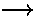rmaxrmin) is also the time it takes to go around the Sun (i.e., forto increase by 2).